# Median, Mode, Skewness, and Kurtosis in MS Access

Published on
63,272 Points
47,872 Views
29 Endorsements
Awarded
Editor's Choice
Community Pick
by Patrick G. Matthews

## Introduction

Like other database applications, Access provides support for computing basic descriptive statistics for a data set, offering such aggregate functions as Count, Sum, Min, Max, standard deviation and variance (StDev, StDevP, Var, and VarP), and average (Avg).  In addition to these typical aggregate functions, Access also offers the so-called "domain aggregate" counterparts for each of the aggregates already mentioned.

That said, there are other useful descriptive statistics for data sets that Access does not support via native functions.  This article will describe how to compute four additional descriptive statistics in Microsoft Access:
Median
Mode
Skewness
Kurtosis

In the next four sections below, this article will describe how to compute the median, mode, skewness, and kurtosis statistics in Access, using examples that I replicate in the attached sample files.
Where feasible, I demonstrate how to calculate these statistics using Jet SQL (Access's native SQL dialect).
I also demonstrate using user defined "domain aggregate" functions, developed in Visual Basic for Applications (VBA), to compute these statistics.

In the Source Code section of this article, I include the VBA source code for four user defined "domain aggregate" functions: DMedian, DMode, DSkewness, and DKurtosis.

This article's Sample Files section includes two sample files, demonstrating the techniques described here with a set of sample data.

In the next to last section, I describe how to implement these new functions in your Microsoft Access projects.

Finally, this article is intended to show you how to compute the median, mode, skewness, and kurtosis statistics using Access, and is not meant as a primer in the statistical constructs or methods themselves.  For readers interested in learning more about the statistics themselves, and how they fit into statistical methods such as hypothesis testing, please see the Further Reading section.

## Median

The median is a measure of central tendency that returns the "middle" value in an ordered set of data.

If there is an odd number of elements in the data set, then the median is that value for which the number of items preceding that value is equal to the number of items following that value: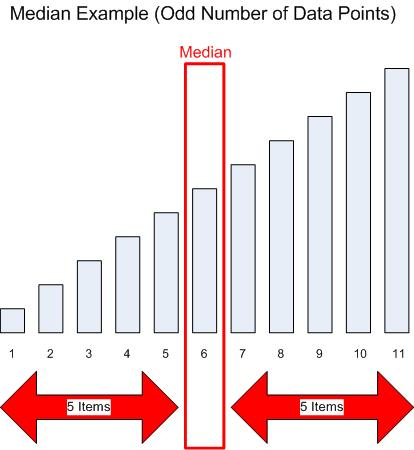If there is an even number of elements, then the median is the simple average of the two middle elements: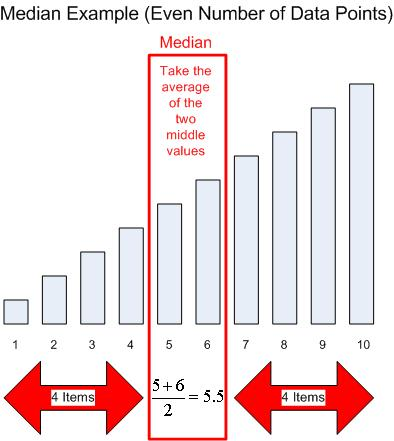In symmetrical distributions, the mean and the median are typically close in value.  However, in skewed distributions, or in samples with extreme outliers, the difference between the mean and the median can be significant.

Access does not provide a native function for determining the median, and writing a SQL query to return the median is a difficult proposition:
In order to calculate a median value using SQL, one must know how many records are in the applicable data set, and often times that result cannot be known without running a query.
Using a sub-query (whether directly in the SQL statement, or by reference as in a "two-step" query) will not work, as the SQL will require a "SELECT TOP N" clause, and Jet cannot accept a variable to define the "N" value.  (Beginning with SQL Server 2005, Transact SQL can use a variable value in a "SELECT TOP N" clause.)
In addition, Jet will often return all of the items in a tie when you use "SELECT TOP N", thus making it difficult to use subqueries to find the median even if you knew the total number of rows ahead of time

Given these limitations, it is simply not feasible to rely on a pure SQL approach to calculate a median, and a VBA approach is preferred.  For this purpose, I created the DMedian user defined function.  It behaves similarly to Access's native domain aggregates, and takes the following arguments:
Column is the name of the column for which you want to find the median value
Tbl is the name of the table or query from which your data comes
Criteria (optional) defines any filtering criteria you wish to apply to the data.  Be sure to use single quotes to qualify text values and # to qualify dates in the Criteria string

For the Column and Tbl arguments, I strongly recommended that you place the column/table/query names in square brackets.  This is mandatory if your database object names violate the usual naming rules, such as by including spaces, punctuation, keywords, etc.

The Source Code section to this article contains the VBA source code for the DMedian function, and the sample database provided in the Sample Files section illustrates four use cases for DMedian on a set of sample data.

``````Median for all data, qryUDF_Median_All

SELECT DMedian("Value", "Sample") AS Median;

Returns:
Median
104
``````

``````Median by Code, qryUDF_Median_ByCode

SELECT Sample.Code, DMedian("Value","Sample","[Code] = '" & Code & "'") AS Median
FROM Sample
GROUP BY Sample.Code;

Returns:
Code  Median
A     100
B     110.5
C     101.5
``````

``````Median by Section, qryUDF_Median_BySection

SELECT Sample.Section, DMedian("Value","Sample","[Section] = '" & Section & "'") AS Median
FROM Sample
GROUP BY Sample.Section;

Returns:
Section     Median
Section 1   105.5
Section 2   103
``````

``````Median by Section and Code, qryUDF_Median_BySectionAndCode

SELECT Sample.Section, Sample.Code, DMedian("Value","Sample","[Section] = '" &
[Section] & "' And [Code] = '" & [Code] & "'") AS Median
FROM Sample
GROUP BY Sample.Section, Sample.Code;

Returns:
Section     Code   Median
Section 1   A      100
Section 1   B      112
Section 1   C      100
Section 2   A      100.5
Section 2   B      107
Section 2   C      103
``````

## Mode

The mode is a measure of central tendency that returns the value(s) in the data set that appear most frequently.  For example, in the data set below, the mode is 5: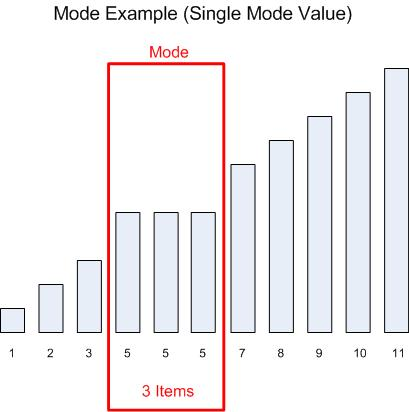In case more than one value is tied for the greatest frequency, the mode is considered the set of all such values.  For example, in this data set, the mode equals 5 and 9: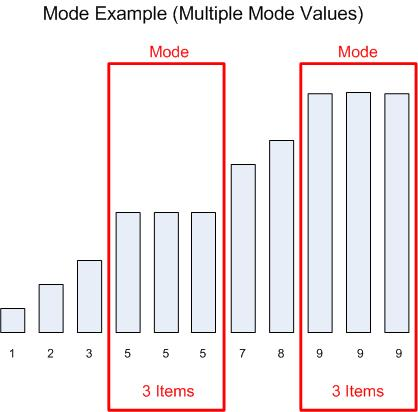Unlike the median, you can use regular SQL quite readily to calculate the mode, by using sub-queries.  However, in cases where there is more than one value in the mode, a pure SQL-based approach will output each modal value on its own row.  If instead you prefer to have all of the modal values in a single column on a single row, you must use VBA to create a user defined function.  You may also find DMode more convenient to use, rather than working out the subquery logic, although the pure SQL approach will execute more quickly.

I created the DMode user defined function fot this purpose.  It behaves similarly to Access's native domain aggregates, and takes the following arguments:
Column is the name of the column for which you want to find the mode value(s)
Tbl is the name of the table or query from which your data comes
Criteria (optional) defines any filtering criteria you wish to apply to the data.  Be sure to use single quotes to qualify text values and # to qualify dates in the Criteria string
ReturnMode (optional) determines which values DMode returns.  If omitted or zero, DMode returns a delimited list of all applicable modal values.  If negative, DMode returns the modal value that is returned first in an ascending sort, and if positive, DMode returns the modal value that is returned first in an descending sort
Delimiter (optional) defines the delimiter to be used if the function is returning more than one modal value.  By default, DMode uses a comma plus a space

For the Column and Tbl arguments, I strongly recommended that you place the column/table/query names in square brackets.  This is mandatory if your database object names violate the usual naming rules, such as by including spaces, punctuation, keywords, etc.

The Source Code section to this article contains the VBA source code for the DMode function, and the sample database provided in the Sample Files section illustrates four use cases each for calculating modes using SQL and using DMedian.

The first four use cases illustrate a pure SQL approach to calculating the modes:

``````Mode for all data, SQL, qrySQL_Mode_All

SELECT TOP 1 Sample.Value
FROM Sample
GROUP BY Sample.Value
ORDER BY Count(Sample.Value) DESC;

Returns:
Value
80
99
``````

``````Mode by Code, SQL, qrySQL_Mode_ByCode

SELECT t1.Code, t1.Value
FROM Sample AS t1
WHERE t1.Value In
(SELECT TOP 1 t2.Value
FROM Sample t2
WHERE t2.Code = t1.Code
GROUP BY t2.Value
ORDER BY Count(t2.Value) Desc)
GROUP BY t1.Code, t1.Value;

Returns:
Code   Value
A      99
A      105
B      115
C      71
``````

``````Mode by Section, SQL, qrySQL_Mode_BySection

SELECT t1.Section, t1.Value
FROM Sample AS t1
WHERE t1.Value In
(SELECT TOP 1 t2.Value
FROM Sample t2
WHERE t2.Section = t1.Section
GROUP BY t2.Value
ORDER BY Count(t2.Value) Desc)
GROUP BY t1.Section, t1.Value;

Returns:
Section     Value
Section 1   80
Section 1   97
Section 1   99
Section 1   108
Section 2   104
Section 2   131
Section 2   140
``````

``````Mode by Section and Code, SQL, qrySQL_Mode_BySectionAndCode

SELECT t1.Section, t1.Code, t1.Value
FROM Sample AS t1
WHERE t1.Value In
(SELECT TOP 1 t2.Value
FROM Sample t2
WHERE t2.Section = t1.Section And t2.Code = t1.Code
GROUP BY t2.Value
ORDER BY Count(t2.Value) Desc)
GROUP BY t1.Section, t1.Code, t1.Value;

Returns:
Section     Code   Value
Section 1   A      99
Section 1   B      95
Section 1   B      114
Section 1   B      115
Section 1   B      116
Section 1   C      81
Section 1   C      108
Section 2   A      105
Section 2   B      123
Section 2   B      131
Section 2   B      140
Section 2   C      104
``````

The next four use cases all demonstrate use of the DMode function to simplify the SQL statements:

``````Mode for all data, UDF, qryUDF_Mode_All

SELECT DMode("Value","Sample") AS ModeAll, DMode("Value","Sample","",-1) AS ModeFirst,
DMode("Value","Sample","",1) AS ModeLast;

Returns:
ModeAll   ModeFirst   ModeLast
80, 99    80          99
``````

``````Mode by Code, UDF, qryUDF_Mode_ByCode

SELECT Sample.Code, DMode("Value","Sample","[Code] = '" & [Code] & "'") AS ModeAll,
DMode("Value","Sample","[Code] = '" & Code & "'",-1) AS ModeFirst,
DMode("Value","Sample","[Code] = '" & Code & "'",1) AS ModeLast
FROM Sample
GROUP BY Sample.Code;

Returns:
Code   ModeAll   ModeFirst   ModeLast
A      105, 99   99          105
B      115       115         115
C      71        71          71
``````

``````Mode by Section, UDF, qryUDF_Mode_BySection

SELECT Sample.Section, DMode("Value","Sample","[Section] = '" & [Section] & "'") AS ModeAll,
DMode("Value","Sample","[Section] = '" & [Section] & "'",-1) AS ModeFirst,
DMode("Value","Sample","[Section] = '" & [Section] & "'",1) AS ModeLast
FROM Sample
GROUP BY Sample.Section;

Returns:
Section     ModeAll           ModeFirst   ModeLast
Section 1   80, 97, 99, 108   80          108
Section 2   104, 131, 140     104         140
``````

``````Mode by Section and Code, UDF, qryUDF_Mode_BySectionAndCode

SELECT Sample.Section, Sample.Code,
DMode("Value","Sample","[Section] = '" & [Section] & "' And [Code] = '" & [Code] & "'") AS ModeAll,
DMode("Value","Sample","[Section] = '" & [Section] & "' And [Code] = '" & [Code] & "'",-1) AS ModeFirst,
DMode("Value","Sample","[Section] = '" & [Section] & "' And [Code] = '" & [Code] & "'",1) AS ModeLast
FROM Sample
GROUP BY Sample.Section, Sample.Code;

Returns:
Section     Code   ModeAll             ModeFirst   ModeLast
Section 1   A      99                  99          99
Section 1   B      95, 114, 115, 116   95          116
Section 1   C      81, 108             81          108
Section 2   A      105                 105         105
Section 2   B      123, 140, 131       123         140
Section 2   C      104                 104         104
``````

## Skewness and Kurtosis

Skewness and kurtosis describe the shape of your data set's distribution.  Skewness indicates how symmetrical the data set is, while kurtosis indicates how heavy your data set is about its mean compared to its tails.  Perfectly symmetrical data sets will have a skewness of zero, and a normally distributed data set will have a kurtosis of approximately three.

For example, this histogram (with an overlay of what a perfect normal distribution would have been) represents the total sample set available in the Access database included with this article: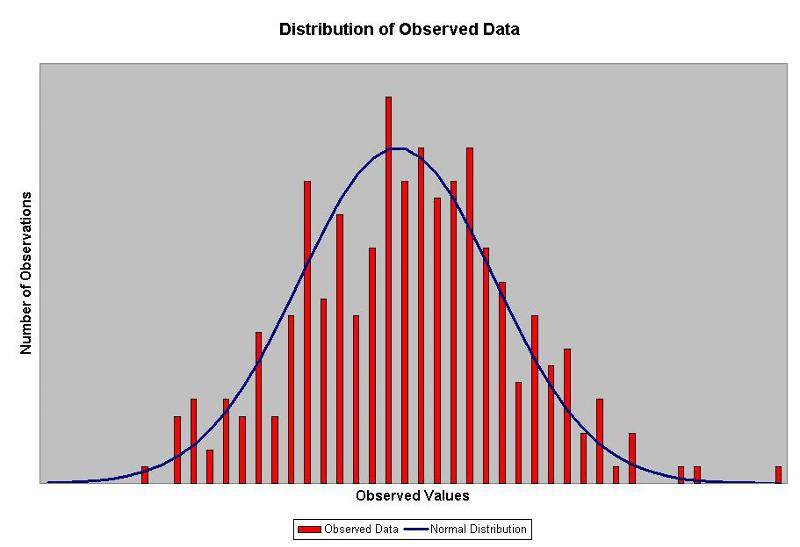As we can see, the sample set is reasonably symmetrical, and the overall distribution appears to be close to a normal distribution, and thus we would expect to find a skewness of close to zero, and a kurtosis of close to three.

(Note: While a normal distribution has kurtosis ˜ 3, other distributions can have a kurtosis of 3, and thus you cannot use kurtosis alone to test the likelihood that your sample was drawn from a normal distribution.  Statisticians have devised numerous other normality tests.)

Data sets are sometimes asymmetrical.  For example, for a left-skewed data set, the median will be lower than the mean, and the skewness will be negative, as seen in this chart: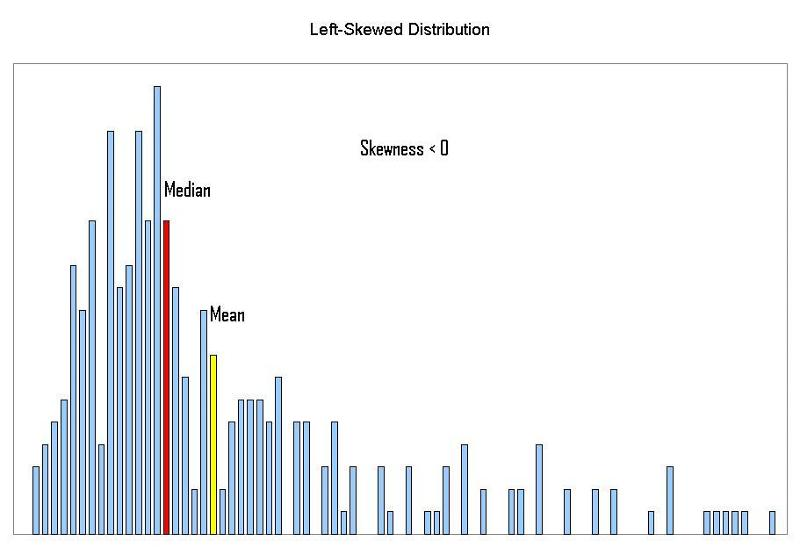In right-skewed data sets, the median is greater than the mean, and the skewness is positive: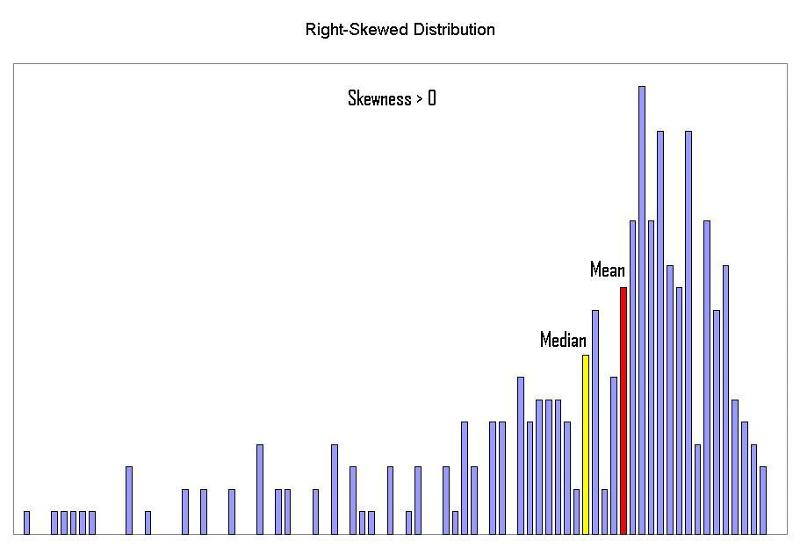In a high kurtosis distribution (kurtosis > 3), data will be clustered much more about the mean, and the tails will be relatively lighter.  The chart below shows a distribution with a relatively high kurtosis (5.3); this distribution has a high proportion of its data points clustered about the mean, and the tails are very light: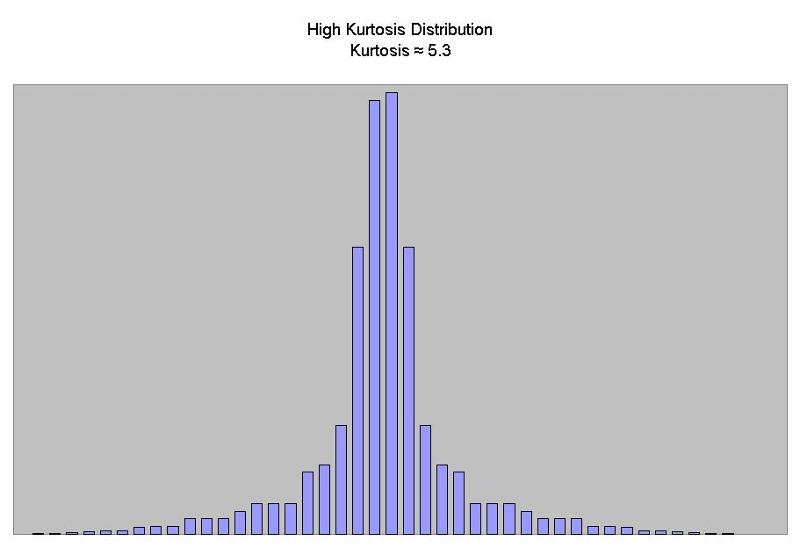Among the commonly-encountered probability distributions described in statistics, the Bernoulli distribution (for probability close to 0% or 100%), Laplace distribution and the logistic distribution tend to have high kurtosis values.

In a low kurtosis distribution (kurtosis < 3), data will not have a pronounced peak about the mean, and will consequently have heavier tails, The chart below shows a distribution with a relatively low kurtosis (1.5); this distribution has relatively few data points clustered about the mean, and the tails are very heavy: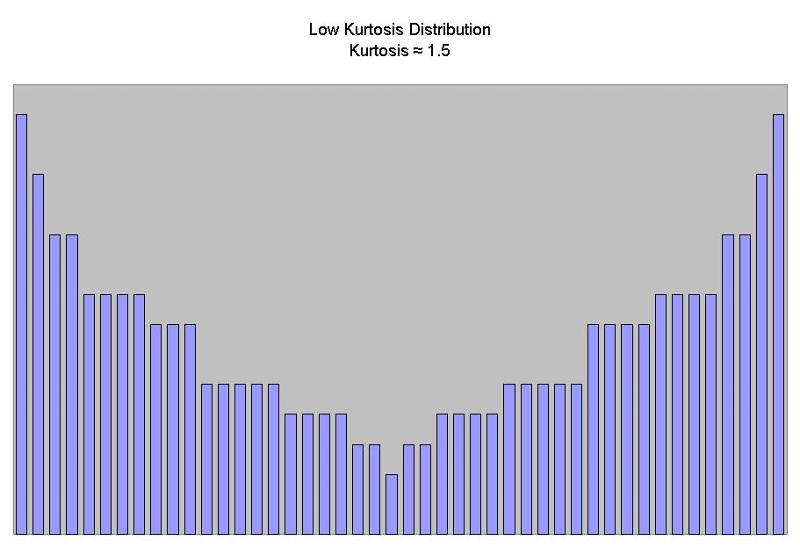Examples of probability distributions with low kurtosis values include the Bernoulli distribution (for probability close to 50%; p = 50% generates the lowest possible kurtosis value, 1), and the discrete uniform distribution.

The formulas for skewness and kurtosis are as follows: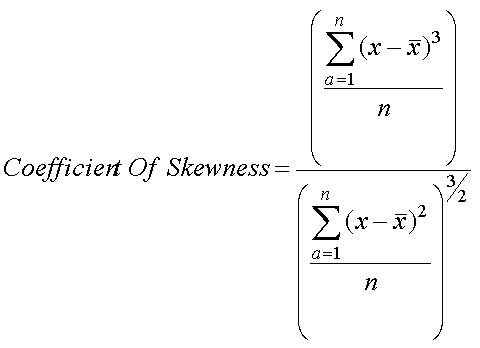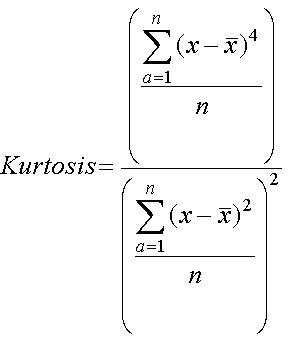You can compute both the skewness and the kurtosis using on SQL.  However, as the following use cases demonstrate, the formulas are very complex.

``````Skewness and kurtosis for all data, SQL, qrySQL_SkewKurt_All

SELECT (Sum((t2.Value-z.TheAvg)^3)/Count(t2.Value))/(Sum((t2.Value-z.TheAvg)^2)/Count(t2.Value))^1.5 AS Skewness,
(Sum((t2.Value-z.TheAvg)^4)/Count(t2.Value))/(Sum((t2.Value-z.TheAvg)^2)/Count(t2.Value))^2 AS Kurtosis
FROM Sample AS t2,
[SELECT Avg(t1.Value) AS TheAvg
FROM Sample t1]. AS z
HAVING Count(t2.Value) > 0;

Returns:
Skewness   Kurtosis
0.0672     3.0465
``````

``````Skewness and kurtosis by Code, SQL, qrySQL_SkewKurt_ByCode

SELECT t2.Code,
(Sum((t2.Value-z.TheAvg)^3)/Count(t2.Value))/(Sum((t2.Value-z.TheAvg)^2)/Count(t2.Value))^1.5 AS Skewness,
(Sum((t2.Value-z.TheAvg)^4)/Count(t2.Value))/(Sum((t2.Value-z.TheAvg)^2)/Count(t2.Value))^2 AS Kurtosis
FROM Sample AS t2 INNER JOIN
[SELECT t1.Code, Avg(t1.Value) AS TheAvg
FROM Sample t1 GROUP BY t1.Code]. AS z ON t2.Code = z.Code
GROUP BY t2.Code
HAVING Count(t2.Value) > 0;

Returns:
Code   Skewness   Kurtosis
A      -0.0289    2.7148
B       0.1870    3.3675
C      -0.0492    2.7303
``````

``````Skewness and kurtosis by Section, SQL, qrySQL_SkewKurt_BySection

SELECT t2.Section,
(Sum((t2.Value-z.TheAvg)^3)/Count(t2.Value))/(Sum((t2.Value-z.TheAvg)^2)/Count(t2.Value))^1.5 AS Skewness,
(Sum((t2.Value-z.TheAvg)^4)/Count(t2.Value))/(Sum((t2.Value-z.TheAvg)^2)/Count(t2.Value))^2 AS Kurtosis
FROM Sample AS t2 INNER JOIN
[SELECT t1.Section, Avg(t1.Value) AS TheAvg
FROM Sample t1 GROUP BY t1.Section]. AS z ON t2.Section = z.Section
GROUP BY t2.Section
HAVING Count(t2.Value) > 0;

Returns:
Section     Skewness   Kurtosis
Section 1   0.0605    3.0673
Section 2   0.0670    2.9209
``````

``````Skewness and kurtosis by Section and Code, SQL, qrySQL_SkewKurt_BySectionAndCode

SELECT t2.Section, t2.Code,
(Sum((t2.Value-z.TheAvg)^3)/Count(t2.Value))/(Sum((t2.Value-z.TheAvg)^2)/Count(t2.Value))^1.5 AS Skewness,
(Sum((t2.Value-z.TheAvg)^4)/Count(t2.Value))/(Sum((t2.Value-z.TheAvg)^2)/Count(t2.Value))^2 AS Kurtosis
FROM Sample AS t2 INNER JOIN
[SELECT t1.Section, t1.Code, Avg(t1.Value) AS TheAvg
FROM Sample t1 GROUP BY t1.Section, t1.Code]. AS z ON (t2.Section = z.Section) AND (t2.Code = z.Code)
GROUP BY t2.Section, t2.Code
HAVING Count(t2.Value) > 0;

Returns:
Section     Code   Skewness   Kurtosis
Section 1   A      -0.0673    2.6510
Section 1   B       0.1870    3.5337
Section 1   C       0.0235    2.5559
Section 2   A       0.0723    2.2061
Section 2   B       0.1778    2.8530
Section 2   C      -0.1484    2.9293
``````

For convenience, I created two user defined "domain aggregate" functions to compute these statistics, DSkewness and DKurtosis.  Both functions take the following arguments:
Column is the name of the column for which you want to find the skewness or kurtosis value
Tbl is the name of the table or query from which your data comes
Criteria (optional) defines any filtering criteria you wish to apply to the data.  Be sure to use single quotes to qualify text values and # to qualify dates in the Criteria string

For the Column and Tbl arguments, I strongly recommended that you place the column/table/query names in square brackets.  This is mandatory if your database object names violate the usual naming rules, such as by including spaces, punctuation, keywords, etc.

The Source Code section to this article contains the VBA source code for the DSkewness and DKurtosis functions, and the sample database provided in the Sample Files section illustrates all four of the use cases described below.

The next four use cases all demonstrate use of the DSkewness and DKurtosis functions to simplify the SQL statements:

``````Skewness and kurtosis for all data, UDF, qryUDF_SkewKurt_All

SELECT DSkewness("Value", "Sample") AS Skewness, DKurtosis("Value", "Sample") AS Kurtosis;

Returns:
Skewness   Kurtosis
0.0672     3.0465
``````

``````Skewness and kurtosis by Code, UDF, qryUDF_SkewKurt_ByCode

SELECT Sample.Code, DSkewness("Value","Sample","[Code] = '" & Code & "'") AS Skewness,
DKurtosis("Value","Sample","[Code] = '" & [Code] & "'") AS Kurtosiss
FROM Sample
GROUP BY Sample.Code;

Returns:
Code   Skewness   Kurtosis
A      -0.0289    2.7148
B       0.1870    3.3675
C      -0.0492    2.7303
``````

``````Skewness and kurtosis by Section, UDF, qryUDF_SkewKurt_BySection

SELECT Sample.Section, DSkewness("Value","Sample","[Section] = '" & [Section] & "'") AS Skewness,
DKurtosis("Value","Sample","[Section] = '" & [Section] & "'") AS Kurtosiss
FROM Sample
GROUP BY Sample.Section;

Returns:
Section     Skewness   Kurtosis
Section 1   0.0605    3.0673
Section 2   0.0670    2.9209
``````

``````Skewness and kurtosis by Section and Code, UDF, qryUDF_SkewKurt_BySectionAndCode

SELECT Sample.Section, Sample.Code,
DSkewness("Value","Sample","[Section] = '" & [Section] & "' And [Code] = '" & [Code] & "'") AS Skewness,
DKurtosis("Value","Sample","[Section] = '" & [Section] & "' And [Code] = '" & [Code] & "'") AS Kurtosiss
FROM Sample
GROUP BY Sample.Section, Sample.Code;

Returns:
Section     Code   Skewness   Kurtosis
Section 1   A      -0.0673    2.6510
Section 1   B       0.1870    3.5337
Section 1   C       0.0235    2.5559
Section 2   A       0.0723    2.2061
Section 2   B       0.1778    2.8530
Section 2   C      -0.1484    2.9293
``````

## Source Code

Below please find the VBA source code for the DMedian, DMode, DSkewness, and DKurtosis functions.

``````Function DMedian(Column As String, Tbl As String, Optional Criteria As String = "")

' Function by Patrick G. Matthews

' Feel free to use and distribute this code, so long as you credit authorship and indicate the
' URL where you found it

' This function calculates the median for a specified column from a specified table/query,
' applying filtering as defined in the Criteria argument.  It is intended for use in Microsoft
' Access.  This function requires a reference to the Microsoft DAO library.

' Records with null values in the specified column are ignored

' This function is labeled according to the domain aggregate function naming convention as it
' behaves similarly to the other domain aggregates

' Column is the column for which you want to determine the median
' Tbl is the source table or query for the data
' Criteria defines any filtering criteria you wish to apply to the data set.  Be sure to enclose
'       text items in single quotes and date values in the # date qualifiers

Dim rs As DAO.Recordset
Dim SQL As String
Dim Count As Long
Dim ValueNum As Long
Dim Counter As Long

Static Alias As String

On Error GoTo ErrHandler

' The subquery needs an alias.  By creating a random string of 20 characters, it is virtually impossible
' that the alias will duplicate a table name already used in the function call.  Since there is no need
' to keep recreating this random string, it is a static variable and thus retains its state between calls

If Alias = "" Then
Randomize
' Build 20-character string using A-Z
For Counter = 1 To 20
Alias = Alias & Chr(65 + Int(Rnd * 26))
Next
End If

' Initialize return value to null

DMedian = Null

' Determine how many records exist for the set we calculate a median for

SQL = "SELECT Count(1) AS TheCount " & _
"FROM " & Tbl & " " & _
"WHERE " & IIf(Trim(Criteria) <> "", Criteria & " And ", "") & Column & " Is Not Null"

Set rs = CurrentDb.OpenRecordset(SQL, dbOpenSnapshot)

Count = rs!TheCount

' ValueNum helps us determine where the "middle" of the set is

ValueNum = Int(Count / 2) + 1

rs.Close

' For an odd number of records, grabbing the median is simple using a subquery.  The subquery
' returns the top N records, and then the main query re-sorts that set in descending order
' and returns the top-most  record

If Count > 0 And (Count Mod 2) = 1 Then
SQL = "SELECT TOP 1 " & Alias & "." & Column & " AS Median " & _
"FROM " & _
"(SELECT TOP " & ValueNum & " " & Column & " " & _
"FROM " & Tbl & " " & _
"WHERE " & IIf(Trim(Criteria) <> "", Criteria & " And ", "") & Column & " Is Not Null " & _
"ORDER BY " & Column & " Asc) AS " & Alias & " " & _
"ORDER BY " & Alias & "." & Column & " Desc"
Set rs = CurrentDb.OpenRecordset(SQL, dbOpenSnapshot)
DMedian = rs!median
rs.Close

' For an even number of records, we have a problem.  We need to average the two middle values,
' but the subquery approach will not work because in a "SELECT TOP N" query Access returns
' ties.  Instead, initialize DMedian to 0, and step through the recordset.  Add together the
' values from the two middle records, and then divide by two for the answer

ElseIf Count > 0 Then
SQL = "SELECT TOP " & ValueNum & " " & Column & " " & _
"FROM " & Tbl & " " & _
"WHERE " & IIf(Trim(Criteria) <> "", Criteria & " And ", "") & Column & " Is Not Null " & _
"ORDER BY " & Column & " Asc"
Set rs = CurrentDb.OpenRecordset(SQL, dbOpenSnapshot)
Counter = 1
DMedian = 0
Do Until Counter > ValueNum Or rs.EOF
If Counter = (ValueNum - 1) Or Counter = ValueNum Then
DMedian = DMedian + rs.Fields(0).Value
End If
rs.MoveNext
Counter = Counter + 1
Loop
rs.Close
DMedian = DMedian / 2
End If

GoTo Cleanup

ErrHandler:
DMedian = CVErr(Err.Number)

Cleanup:
Set rs = Nothing

End Function
``````

``````Function DMode(Column As String, Tbl As String, Optional Criteria As String = "", _
Optional ReturnMode As Long = 0, Optional Delimiter As String = ", ")

' Function by Patrick G. Matthews

' Feel free to use and distribute this code, so long as you credit authorship and indicate the
' URL where you found it

' This function calculates the mode for a specified column from a specified table/query,
' applying filtering as defined in the Criteria argument.  It is intended for use in Microsoft
' Access.  This function requires a reference to the Microsoft DAO library.

' Records with null values in the specified column are ignored

' This function is labeled according to the domain aggregate function naming convention as it
' behaves similarly to the other domain aggregates

' Column is the column for which you want to determine the median
' Tbl is the source table or query for the data
' Criteria defines any filtering criteria you wish to apply to the data set.  Be sure to enclose
'       text items in single quotes and date values in the # date qualifiers
' ReturnMode indicates which modal value is returned.
'       If 0 or omitted, all modal values are returned in a delimited list
'       If negative, returns the modal value returned first in an ascending sort
'       If positive, returns the modal value returned first in a descending sort
' Delimiter is the delimiter used in making a list of modal values.  Default is ,+space

Dim rs As DAO.Recordset
Dim SQL As String

On Error GoTo ErrHandler

' Build up the SQL statement, then open recordset

SQL = "SELECT TOP 1 " & Column & " " & _
"FROM " & Tbl & " " & _
"WHERE " & IIf(Trim(Criteria) <> "", Criteria & " And ", "") & Column & " Is Not Null " & _
"GROUP BY " & Column & " " & _
"ORDER BY Count(1) Desc" & Switch(ReturnMode = 0, "", ReturnMode < 0, ", " & Column & " Asc", _
ReturnMode > 0, ", " & Column & " Desc")

Set rs = CurrentDb.OpenRecordset(SQL, dbOpenSnapshot)

' If ReturnMode = 0, function returns delimited list of all modal values

If ReturnMode = 0 Then
DMode = ""
Do Until rs.EOF
DMode = DMode & Delimiter & rs.Fields(0).Value
rs.MoveNext
Loop
DMode = Mid(DMode, Len(Delimiter) + 1)

' If ReturnMode is negative, function returns the modal value that sorts first ascending, and if
' ReturnMode is positive, function returns the modal value that sorts first descending

Else
DMode = rs.Fields(0).Value
End If

GoTo Cleanup

ErrHandler:
DMode = CVErr(Err.Number)

Cleanup:
Set rs = Nothing

End Function
``````

``````Function DSkewness(Column As String, Tbl As String, Optional Criteria As String = "")

' Function by Patrick G. Matthews

' Feel free to use and distribute this code, so long as you credit authorship and indicate the
' URL where you found it

' This function calculates the sample skewness for a specified column from a specified
' table/query, applying filtering as defined in the Criteria argument.  It is intended for use
' in Microsoft Access.  This function requires a reference to the Microsoft DAO library.

' Records with null values in the specified column are ignored

' This function is labeled according to the domain aggregate function naming convention as it
' behaves similarly to the other domain aggregates

' Column is the column for which you want to determine the median
' Tbl is the source table or query for the data
' Criteria defines any filtering criteria you wish to apply to the data set.  Be sure to enclose
'       text items in single quotes and date values in the # date qualifiers

Dim rs As DAO.Recordset
Dim SQL As String
Dim Counter As Long

Static Alias As String

On Error GoTo ErrHandler

' The subquery needs an alias.  By creating a random string of 20 characters, it is virtually impossible
' that the alias will duplicate a table name already used in the function call.  Since there is no need
' to keep recreating this random string, it is a static variable and thus retains its state between calls

If Alias = "" Then
Randomize
' Build 20-character string using A-Z
For Counter = 1 To 20
Alias = Alias & Chr(65 + Int(Rnd * 26))
Next
End If

' Build up SQL statement

SQL = "SELECT (Sum((" & Column & " - " & Alias & ".TheAvg) ^ 3) / Count(" & Column & ")) / " & _
"(Sum((" & Column & " - " & Alias & ".TheAvg) ^ 2) / Count(" & Column & ")) ^ 1.5 AS Skew " & _
"FROM " & Tbl & ", " & _
"(SELECT Avg(" & Column & ") AS TheAvg, StDev(" & Column & ") AS TheStDev " & _
"FROM " & Tbl & " " & _
"WHERE " & IIf(Trim(Criteria) <> "", Criteria & " And ", "") & Column & " Is Not Null) AS " & Alias & " " & _
"WHERE " & IIf(Trim(Criteria) <> "", Criteria & " And ", "") & Column & " Is Not Null " & _
"HAVING Count(" & Column & ") > 2 and " & Alias & ".TheStDev <> 0"

Set rs = CurrentDb.OpenRecordset(SQL, dbOpenSnapshot)

If Not rs.EOF Then
DSkewness = rs!Skew
Else
DSkewness = Null
End If

rs.Close

GoTo Cleanup

ErrHandler:
DSkewness = CVErr(Err.Number)

Cleanup:
Set rs = Nothing

End Function
``````

``````Function DKurtosis(Column As String, Tbl As String, Optional Criteria As String = "")

' Function by Patrick G. Matthews

' Feel free to use and distribute this code, so long as you credit authorship and indicate the
' URL where you found it

' This function calculates the sample kurtosis for a specified column from a specified
' table/query, applying filtering as defined in the Criteria argument.  It is intended for use
' in Microsoft Access.  This function requires a reference to the Microsoft DAO library.

' Records with null values in the specified column are ignored

' This function is labeled according to the domain aggregate function naming convention as it
' behaves similarly to the other domain aggregates

' Column is the column for which you want to determine the median
' Tbl is the source table or query for the data
' Criteria defines any filtering criteria you wish to apply to the data set.  Be sure to enclose
'       text items in single quotes and date values in the # date qualifiers

Dim rs As DAO.Recordset
Dim SQL As String
Dim Counter As Long

Static Alias As String

On Error GoTo ErrHandler

' The subquery needs an alias.  By creating a random string of 20 characters, it is virtually impossible
' that the alias will duplicate a table name already used in the function call.  Since there is no need
' to keep recreating this random string, it is a static variable and thus retains its state between calls

If Alias = "" Then
Randomize
' Build 20-character string using A-Z
For Counter = 1 To 20
Alias = Alias & Chr(65 + Int(Rnd * 26))
Next
End If

' Build up SQL statement

SQL = "SELECT (Sum((" & Column & " - " & Alias & ".TheAvg) ^ 4) / Count(" & Column & ")) / " & _
"(Sum((" & Column & " - " & Alias & ".TheAvg) ^ 2) / Count(" & Column & ")) ^ 2 AS Skew " & _
"FROM " & Tbl & ", " & _
"(SELECT Avg(" & Column & ") AS TheAvg, StDev(" & Column & ") AS TheStDev " & _
"FROM " & Tbl & " " & _
"WHERE " & IIf(Trim(Criteria) <> "", Criteria & " And ", "") & Column & " Is Not Null) AS " & Alias & " " & _
"WHERE " & IIf(Trim(Criteria) <> "", Criteria & " And ", "") & Column & " Is Not Null " & _
"HAVING Count(" & Column & ") > 3 and " & Alias & ".TheStDev <> 0"

Set rs = CurrentDb.OpenRecordset(SQL, dbOpenSnapshot)

If Not rs.EOF Then
DKurtosis = rs!Skew
Else
DKurtosis = Null
End If

rs.Close

GoTo Cleanup

ErrHandler:
DKurtosis = CVErr(Err.Number)

Cleanup:
Set rs = Nothing

End Function
``````

## Sample Files

Access database
Single table, "Sample", containing the data used for example queries and results in this article
VBA source code for the DMedian, DMode, DSkewness, and DKurtosis functions

Stats-Part-1.mdb

Excel workbook
Single worksheet containing the same data as in the Access database
Sample statistics for the whole data set plus subsets corresponding to those in Access
Note: Excel's kurtosis calculation is scaled differently than the kurtosis calculated in the examples in this article and in the Access database.  Excel reports the "excess kurtosis", for which a normal distribution would have a value of approximately zero

Stats-Article-Part-1.xls

## Implementing DMedian, DMode, DSkewness, and DKurtosis in Your Access Projects

To implement the DMedian, DMode, DSkewness, and/or DKurtosis functions in your Access projects, simply copy the source code listed for the desired functions(s) in this article, and paste the code into a regular VBA module in your Access project.

Once you have added the code to your Access project, you will be able to use the functions you added in queries, forms, reports, and in other VBA procedures in your project.

Notes:
To reach the Visual Basic editor from Access, use Alt+F11
Be sure to post the code in a "regular" module, and not a class module, form module, or report module
Make sure that the module name does not match any of the sub or function names in that module

As noted in the introduction, the focus of this article in on calculating the median, mode, skewness, and kurtosis statistics using Access, and thus I assume readers will have at least a working knowledge of what these statistics are and how to use them.

Readers wishing to learn more about these statistics, and statistical methods generally, may want to start a good general purpose introduction to probability and statistics.  The following books generally get good reviews as introductory texts for people without advanced math backgrounds who need to understand basic statistics:
Statistics Without Tears: A Primer for Non-Mathematicians, by Derek Rowntree
Statistics for Dummies, by Deborah Rumsey
The Complete Idiot's Guide to Statistics, by Robert A. Donnelly

For people looking for a more rigorous treatment of statistics, you may want to pick up a used copy of a textbook from a college introductory level "Intro to Stats" course.  I would have recommended my own textbook from back in the day, which I still refer to often, except that the latest edition is getting horrendous reviews.

For quick definitions and explanation of terms, it is difficult to beat Wikipedia.  The following articles provide explanations for many of the statistical terms mentioned in this article:
The statistical measures mentioned in this article: Median, Mode, Skewness, and Kurtosis
Hypothesis testing in general

Other sites that may be useful:

Beyond these, any search on "introduction to statistics" or "hypothesis" testing" on the major search engines will return thousands of hits.

=-=-=-=-=-=-=-=-=-=-=-=-=-=-=-=-=-=-=-=-=-=-=-=-=-=-=-=-=-=-=-=-=-=-=-=-=-=-=-=-=